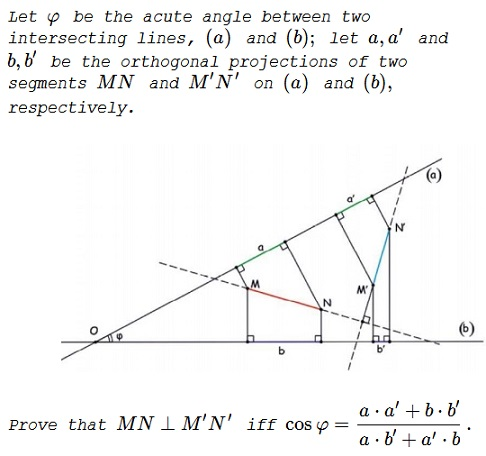# Stathis Koutras' Theorem II

### Problem### Solution 1

Choose $O=(0,0),\,$ $OA:\,y=0\,$ $OC:\, y=mx,\,$ with $m\gt 0;\,$ $A=(u,0),\,$ $u\gt 0;\,$ $C=(v,mv),\,$ $v\gt 0;\,$ $B=(u+b,0);\,$ $b\gt 0;\,$ $D=\displaystyle\left(v+\frac{a}{\sqrt{1+m^2}},m\left(v+\frac{a}{\sqrt{1+m^2}}\right)\right).$Clearly, $MA:\,x=u\,$ and $MC:\,x+my=v(1+m^2),\,$ implying $M=\displaystyle\left(u,\frac{v(1+m^2)-u}{m}\right).\,$ Analogously, $N=\displaystyle\left(u+b,\frac{v(1+m^2)+a\sqrt{1+m^2}-u-b}{m}\right).\,$ From here, the slope of $MN\,$ is $\displaystyle\frac{a\sqrt{1+m^2}-b}{mb}.\,$ Similarly, the slope of $M'N'\,$ is $\displaystyle\frac{a'\sqrt{1+m^2}-b'}{mb'}.\,$ We have $MN\perp M'N'\,$ is equivalent to

$\displaystyle\frac{a\sqrt{1+m^2}-b}{mb}\cdot\frac{a'\sqrt{1+m^2}-b'}{mb'}=-1$

which is the same as $\displaystyle\frac{aa'+bb'}{ab'+ba'}=\frac{1}{\sqrt{1+m^2}}=\cos\varphi.$

### Solution 2

Let $K,L,P,Q\,$ be points on $(a),(b),\,$ as shown on the diagram below ao that $(OP)=,\,$ $(OQ)=b,\,$ $(OL)=a',\,$ $(OK)=b'.$According to Stathis Koutras' theorem, $MN\perp QP\,$ and $M'N'\perp LK,\,$ so that $MN\perp M'N'\,$ iff $\overrightarrow{QP}\perp \overrightarrow{LK},\,$ which is equivalent to $(\overrightarrow{OP}-\overrightarrow{OQ})\cdot (\overrightarrow{OK}-\overrightarrow{OL})=\overrightarrow{0},\,$ i.e.,

$\overrightarrow{OP}\cdot\overrightarrow{OK}-\overrightarrow{OP}\cdot\overrightarrow{OL}-\overrightarrow{OQ}\cdot\overrightarrow{OK}+\overrightarrow{OQ}\cdot\overrightarrow{OL}=\overrightarrow{0}.$

Since $\overrightarrow{OP}\,$ and $\overrightarrow{OL}\,$ have opposite directions (as do $\overrightarrow{OQ}\,$ and $\overrightarrow{OK}),\,$ the above is equivalent to

$|\overrightarrow{OP}|\cdot|\overrightarrow{OK}|\cos (\pi-\varphi)-|\overrightarrow{OP}|\cdot|\overrightarrow{OL}|-|\overrightarrow{OQ}|\cdot|\overrightarrow{OK}|+|\overrightarrow{OQ}|\cdot|\overrightarrow{OL}|\cos (\pi-\varphi)=0.$

This is equivalent to $ab'\cos\varphi+aa'+bb'-a'b\cos\varphi=0,\,$ one step way from the required identity,

### Acknowledgment

Leo Giugiuc has kindly communicated to me another problem of Stathis Koutras, along with his solution. The problem has originally been posted at the Οι Ρομαντικοι της Γεωμετριας (Romantics of Geometry) facebook group. Solution 2 is by Stathis Koutras.